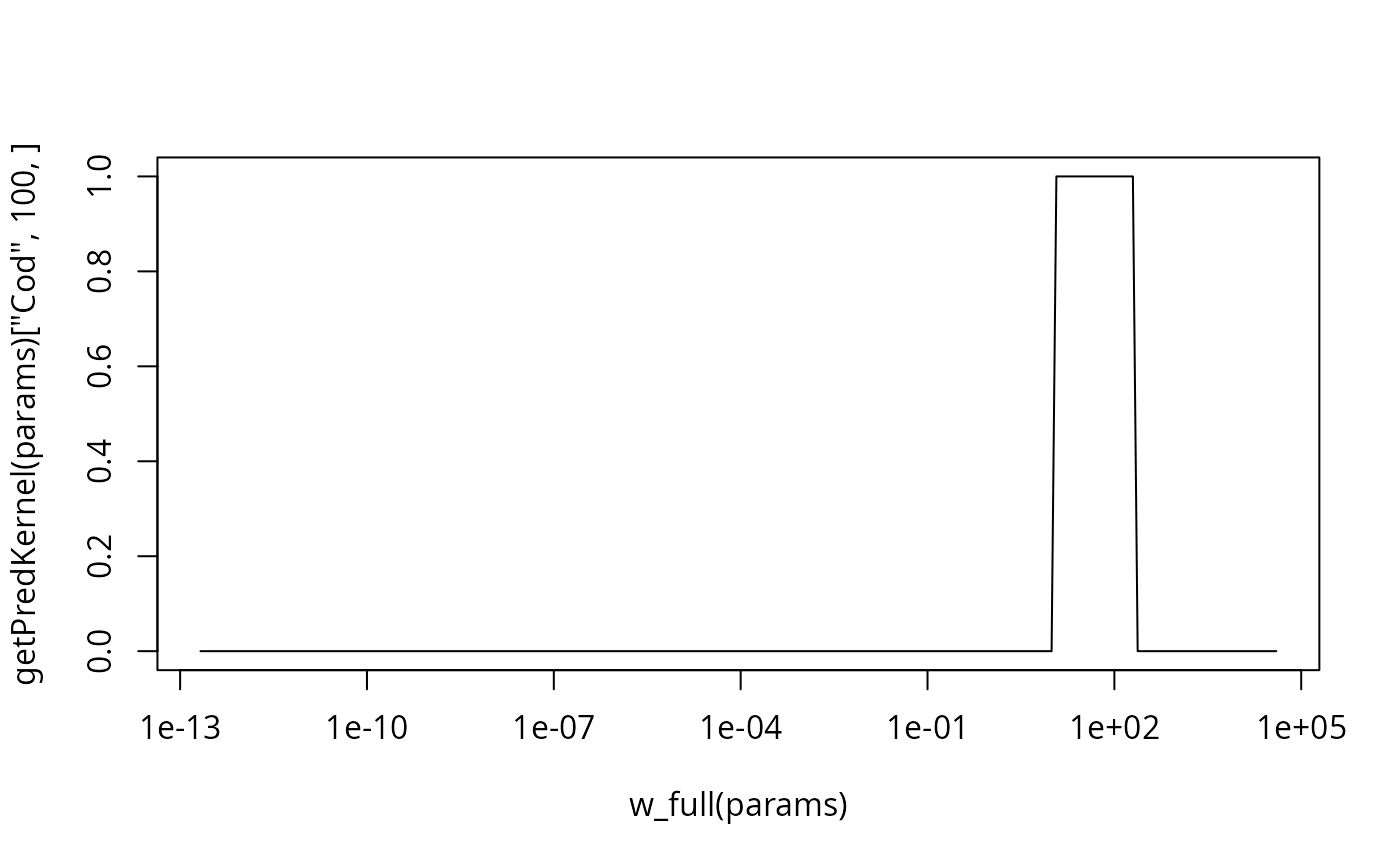The predation kernel determines the distribution of prey sizes that a predator feeds on. It is used in getEncounter() when calculating the rate at which food is encountered and in getPredRate() when calculating the rate at which a prey is predated upon. The predation kernel can be a function of the predator/prey size ratio or it can be a function of the predator size and the prey size separately. Both types can be set up with this function.

## Usage

setPredKernel(params, pred_kernel = NULL, reset = FALSE, ...)

getPredKernel(params)

pred_kernel(params)

pred_kernel(params) <- value

## Arguments

params

A MizerParams object

pred_kernel

Optional. An array (species x predator size x prey size) that holds the predation coefficient of each predator at size on each prey size. If not supplied, a default is set as described in section "Setting predation kernel".

resetIf set to TRUE, then the predation kernel will be reset to the value calculated from the species parameters, even if it was previously overwritten with a custom value. If set to FALSE (default) then a recalculation from the species parameters will take place only if no custom value has been set.

...

Unused

value

pred_kernel

## Value

setPredKernel(): A MizerParams object with updated predation kernel.

getPredKernel() or equivalently pred_kernel(): An array (predator species x predator_size x prey_size)

## Setting predation kernel

Kernel dependent on predator to prey size ratio

If the pred_kernel argument is not supplied, then this function sets a predation kernel that depends only on the ratio of predator mass to prey mass, not on the two masses independently. The shape of that kernel is then determined by the pred_kernel_type column in species_params.

The default for pred_kernel_type is "lognormal". This will call the function lognormal_pred_kernel() to calculate the predation kernel. An alternative pred_kernel type is "box", implemented by the function box_pred_kernel(), and "power_law", implemented by the function power_law_pred_kernel(). These functions require certain species parameters in the species_params data frame. For the lognormal kernel these are beta and sigma, for the box kernel they are ppmr_min and ppmr_max. They are explained in the help pages for the kernel functions. Except for beta and sigma, no defaults are set for these parameters. If they are missing from the species_params data frame then mizer will issue an error message.

You can use any other string for pred_kernel_type. If for example you choose "my" then you need to define a function my_pred_kernel that you can model on the existing functions like lognormal_pred_kernel().

When using a kernel that depends on the predator/prey size ratio only, mizer does not need to store the entire three dimensional array in the MizerParams object. Such an array can be very big when there is a large number of size bins. Instead, mizer only needs to store two two-dimensional arrays that hold Fourier transforms of the feeding kernel function that allow the encounter rate and the predation rate to be calculated very efficiently. However, if you need the full three-dimensional array you can calculate it with the getPredKernel() function.

Kernel dependent on both predator and prey size

If you want to work with a feeding kernel that depends on predator mass and prey mass independently, you can specify the full feeding kernel as a three-dimensional array (predator species x predator size x prey size).

You should use this option only if a kernel dependent only on the predator/prey mass ratio is not appropriate. Using a kernel dependent on predator/prey mass ratio only allows mizer to use fast Fourier transform methods to significantly reduce the running time of simulations.

The order of the predator species in pred_kernel should be the same as the order in the species params dataframe in the params object. If you supply a named array then the function will check the order and warn if it is different.

Other functions for setting parameters: gear_params(), setExtMort(), setFishing(), setInitialValues(), setInteraction(), setMaxIntakeRate(), setMetabolicRate(), setParams(), setReproduction(), setSearchVolume(), species_params()

## Examples

## Set up a MizerParams object
params <-  NS_params

## If you change predation kernel parameters after setting up a model,
#  this will be used to recalculate the kernel
species_params(params)["Cod", "beta"] <- 200

## You can change to a different predation kernel type
species_params(params)$ppmr_max <- 4000 species_params(params)$ppmr_min <- 200
species_params(params)\$pred_kernel_type <- "box"
plot(w_full(params), getPredKernel(params)["Cod", 100, ], type="l", log="x")## If you need a kernel that depends also on prey size you need to define
# it yourself.
pred_kernel <- getPredKernel(params)
pred_kernel["Herring", , ] <- sweep(pred_kernel["Herring", , ], 2,
params@w_full, "*")
params<- setPredKernel(params, pred_kernel = pred_kernel)